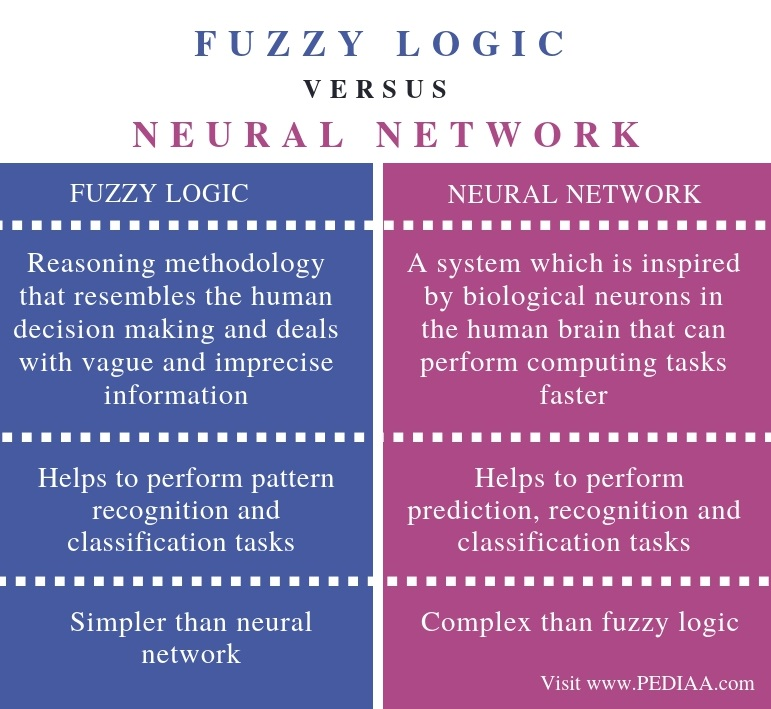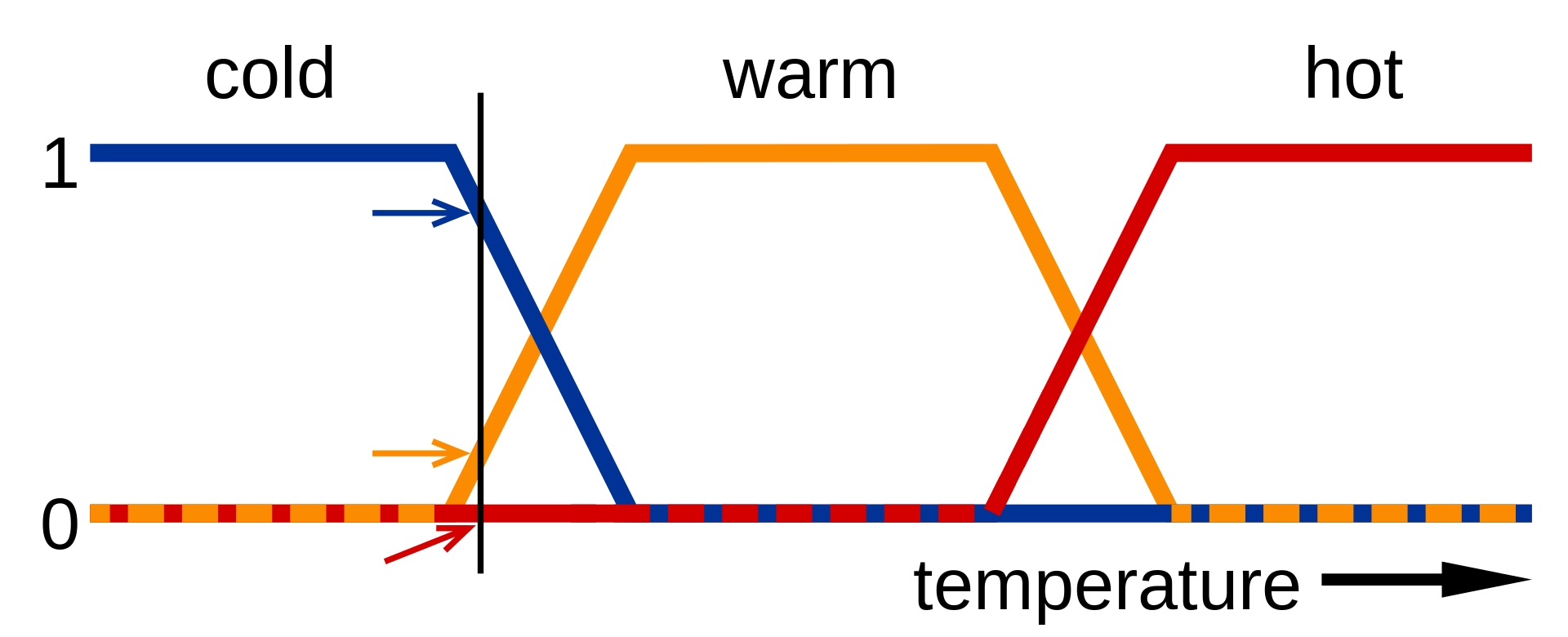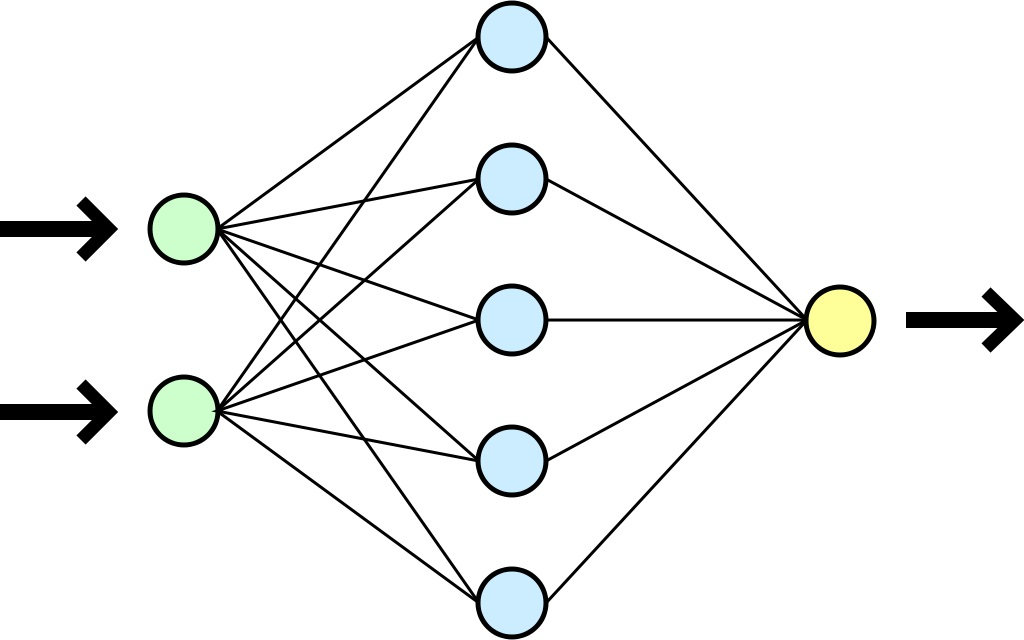# What is the Difference Between Fuzzy Logic and Neural Network

The main difference between fuzzy logic and neural network is that the fuzzy logic is a reasoning method that is similar to human reasoning and decision making, while the neural network is a system that is based on the biological neurons of a human brain to perform computations.

Artificial Intelligence (AI) is the simulation of human intelligence processes by machines; especially, the computer systems. In other words, it gives a machine or a computer the ability to perform tasks similar to a human. Fuzzy logic and neural network are two sub categories of Artificial Intelligence. In brief, these technologies help to build useful applications that can make effective decisions.

### Key Areas Covered

1. What is a Fuzzy Logic
-Definition, Functionality
2. What is a Neural Network
-Definition, Functionality
3. Difference Between Fuzzy Logic and Neural Network
-Comparison of key differences

### Key Terms

Artificial Intelligence, Feedback Network, Feed-forward Network, Fuzzy Logic, Neural Network,
Perceptron## What is a Fuzzy Logic

Fuzzy logic is a reasoning method that is similar to human reasoning. In other words, a fuzzy logic-based system can make decisions similar to a human. It involves all intermediate possibilities between digital values Yes and No. The conventional logic block is capable of obtaining input and generating output as true or false similar, to a human’s yes or no. Microwaves, washing machines, air conditioners and vacuum cleaners are some applications that use fuzzy logic.The main objective of using fuzzy logic is to control machines. It also provides acceptable reasoning. Moreover, it helps to handle uncertainty. It is possible to implement fuzzy logic to any kind of system irrespective of its size.  Furthermore,  the system can consist of hardware, software or a combination of both.

Overall, fuzzy logic is easier to understand. However, there is no systematic approach to design a fuzzy logic-based system. It is also not effective for systems that require higher accuracy.

## What is a Neural Network

A neural network is a network that is similar to a human brain. In other words, a neural network is inspired by biological neurons. There are millions of neurons in the human brain, and the information passes from one neuron to another. A neural network works similar to that and is capable of performing computations faster.The basic type of a neural network is a perceptron. It contains neutrons, and each neuron in it is given an input and has a weight. The neutron computes some function on the weighted inputs and provides the output. Each input is multiplied by the corresponding weight, and the resulting output goes through an activation function to provide the final output. Here, this weight emphasizes the effectiveness of the input. Besides, there is an additional parameter called bias to adjust the weights. Moreover, an activation function converts the inputs to outputs according to a threshold value. Linear, tanh, sigmoid and softmax are some activation functions. It is possible to select an activation function depending on the problem.

Furthermore, there are two types of neural networks as feedforward and feedback. In the feed-forward network, the information passes from input to output, and there is no feedback loop. Meanwhile, in feedback networks, the information passes in both directions, and there is no feedback path.

The feedforward network is further divided into a single layer and multi-layer network. In a single layer network, the input layer connects to the output layer while in a multi-layer network, there are layers in addition to the input and output layers called hidden layers.

## Difference Between Fuzzy Logic and Neural Network

### Definition

Fuzzy logic is a reasoning methodology that resembles human decision making and deals with vague and imprecise information, while a neural network is a system inspired by biological neurons in the human brain and can perform computing tasks faster.

### Usage

Moreover, fuzzy logic helps to perform pattern recognition and classification tasks, while the neural network helps to perform prediction, recognition and classification tasks.

### Complexity

The fuzzy logic is simpler than the neural network.

### Conclusion

In brief, programmers use fuzzy logic and neural networks to develop applications. The main difference between fuzzy logic and neural network is that fuzzy logic is a reasoning method that is similar to human reasoning and decision making, while the neural network is a system that is based on the biological neurons of a human brain to perform computations.

##### References:

1. edureka! Data Science Full Course, YouTube, 17 Mar. 2019, Available here.
2. “Artificial Intelligence – Fuzzy Logic Systems” Tutorialspoint.com, Available here.

##### Image Courtesy:

1.”Fuzzy logic – temperature” By fullofstars – original (gif): Image:Warm fuzzy logic member function.gif (CC BY-SA 3.0) via Commons Wikimedia
2.”A simplified view of an artifical neural network” By Dake, Mysid – Vectorized by Mysid in CorelDraw on an image by Dake (CC BY 1.0) via Commons Wikimedia